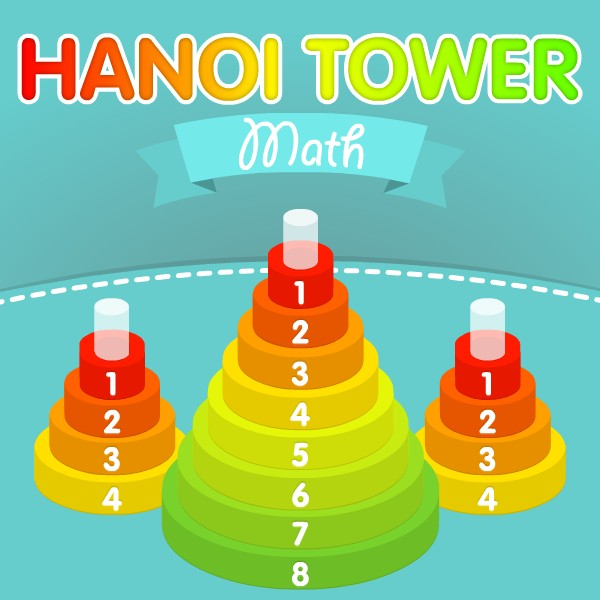# Math Mahjong Relax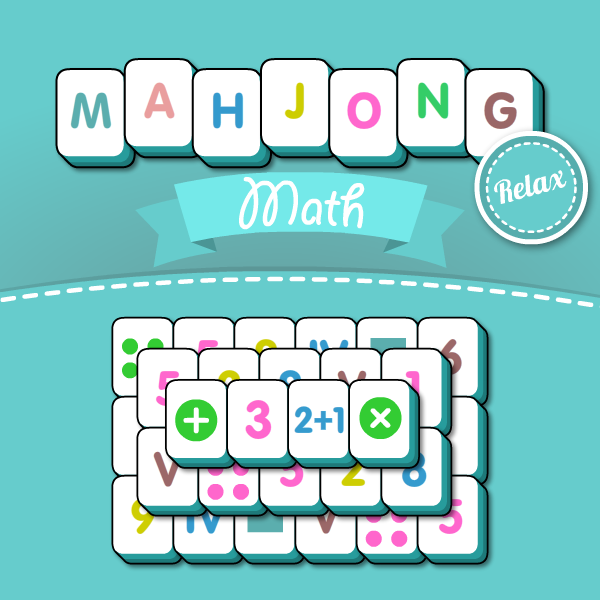• Played:35,573

### Embed Code:

<iframe src="//playcoolmath.com/en/math-games/math-mahjong-relax/play" width="1364px" height="768px" frameborder="0"></iframe>

### Share with Friends:

Welcome to online Math Mahjong Relax. Math Mahjong Relax is a traditional solitaire game where you have to take apart the construction made of dice. The game helps to improve your attention, speed and math skills.

Dice differ in the image on the upper edge. With one move, you can get only two same and open dice. The dice is considered open if its left or right face is open. The game includes 36 levels from simple to complex ones. In the beginner levels you will learn the rules, find out which dice differ and which ones can be interchangeable.

Dice have three suits: numbers, dots, Roman numerals. The game also includes the dice of other types: geometric figures, arithmetic functions, Greek letters (which are most commonly used in math definitions). In more complex levels, we added arithmetic examples about addition and subtraction. You will have to discover the same dice, for example dice 5, 3+2 or 1+4 are the same dice, since their total amount equals 5. Try to pass all the levels and get 3 stars in every level.

•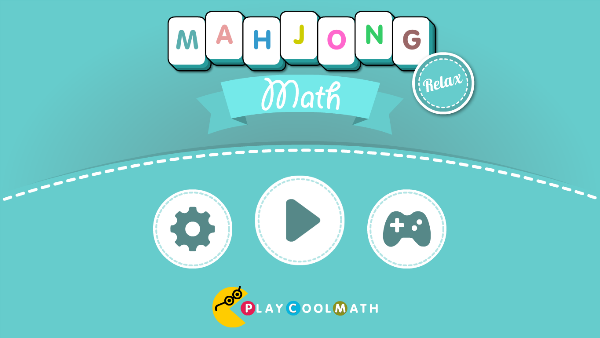•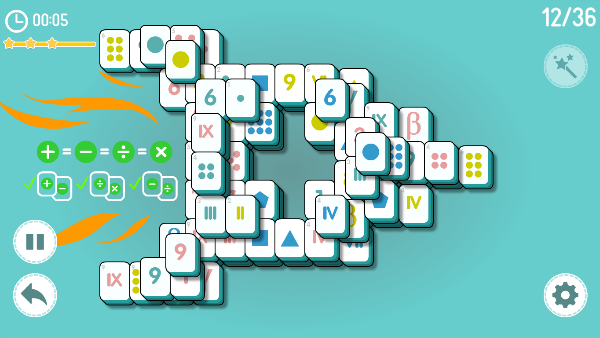•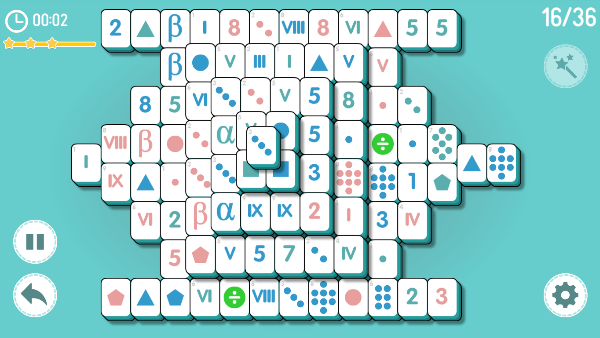•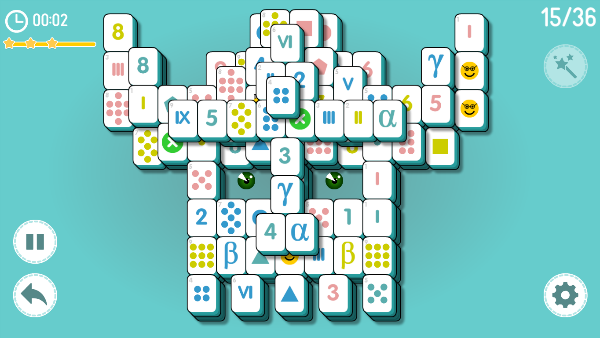•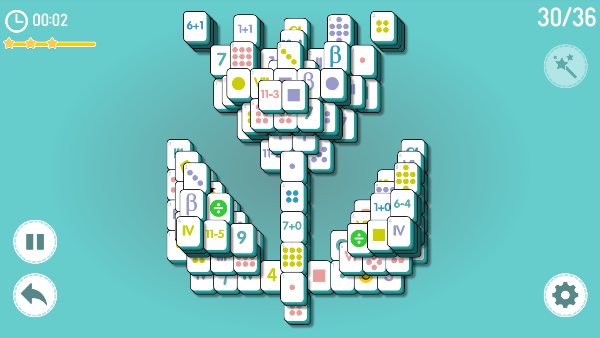•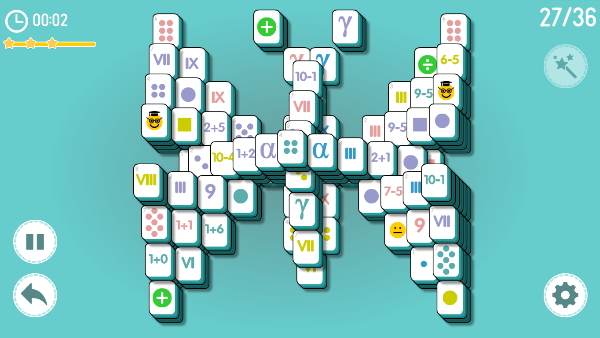•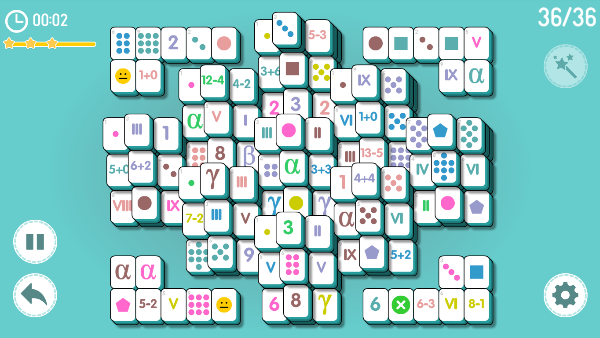•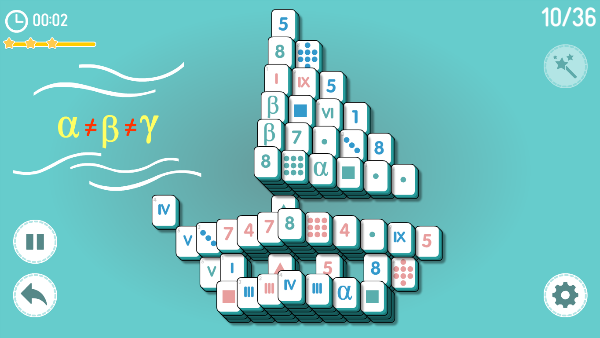## 1024 Math

1024 Math reminds a game of 2048 from Gabriele Cirulli. There are 18 levels in this game. You need to complete all the goals when you get at each level. You can move all the blocks up, down, left or right. You can...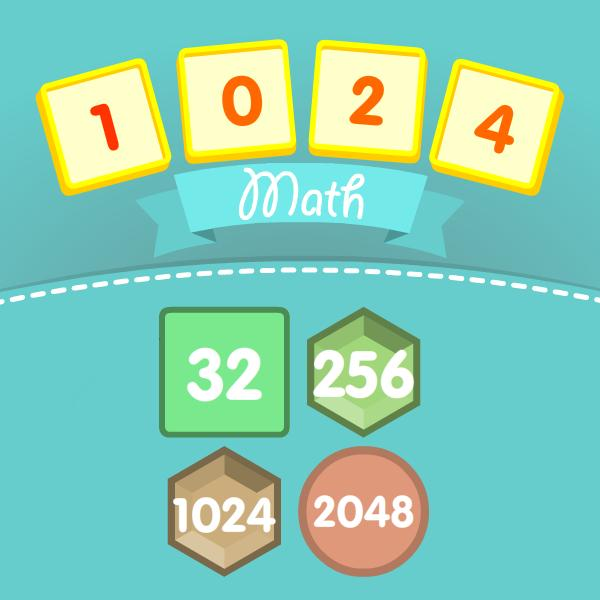## Math Number Challenge

In this game, you need be finding figures for speed. It looks quite easy for the first time. However, at every level new tasks appear complicating the search for numbers. In the game, you will learn figures in the decimal system. You will find out how ...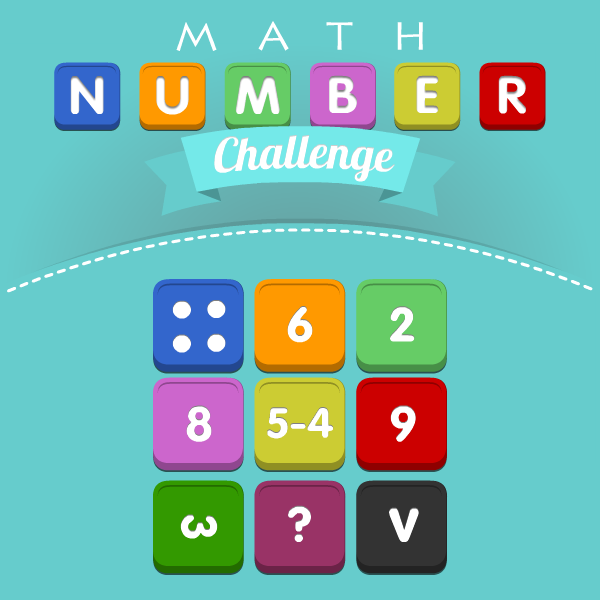## Math Pixel Puzzle

Math pixel puzzle is a very unusual game. The rules are very simple. You will see an image with pixels of various colors. After three seconds, the pixels fly apart in 3D space. Your task is to rotate the space so that ...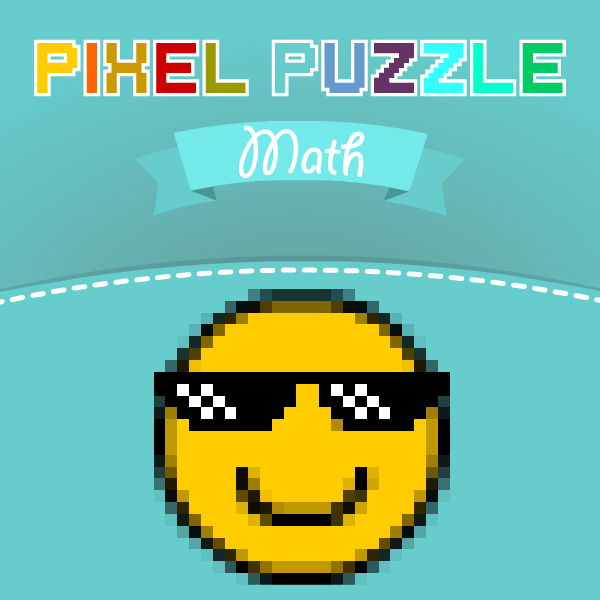## Math Tower of Hanoi

The Tower of Hanoi is a puzzle game invented in 19th century by the French mathematician Eduard Lucas. This guy got inspired by the legend about priests in Hindi castle. Those priests got 3 bars, one ...